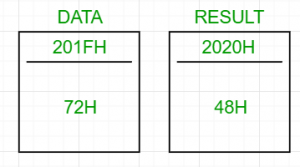# 8085 program to convert a BCD number to binary

Problem – Write an assembly language program for converting a 2 digit BCD number to its binary equivalent using 8085 microprocessor.

Examples:

```Input : 72H (0111 0010)2
Output : 48H (in hexadecimal) (0011 0000)2
((4x16)+(8x1))=72
```Algorithm:

1. Load the BCD number in the accumulator
2. Unpack the 2 digit BCD number into two separate digits. Let the left digit be BCD1 and the right one BCD2
3. Multiply BCD1 by 10 and add BCD2 to it

If the 2 digit BCD number is 72, then its binary equivalent will be
7 x OAH + 2 = 46H + 2 = 48H

Steps:

1. Load the BCD number from the memory location (201FH, arbitrary choice) into the accumulator
2. Temporarily store the accumulator’s value in B
3. Obtain BCD2 by ANDing the accumulator with 0FH and store it in C
4. Restore the original value of the accumulator by moving the value in B to A. AND the accumulator with F0H
5. If the value in the accumulator equals 0, then BCD2 is the final answer and store it in the memory location, 2020H (arbitrary)
6. Else, shift the accumulator to right 4 times to obtain BCD1. Next step is to multiply BCD1 by 0AH
7. Multiplication: Move BCD1 to D and initialise E with 0AH as the counter. Clear the accumulator to 0 and add D to it E number of times
8. Finally, add C to the accumulator and store the result in 2020H

2020H contains the result.

2000H LDA 201FH
2001H
2002H
2003H MOV B, A
2004H ANI 0FH
2005H
2006H MOV C, A
2007H MOV A, B
2008H ANI F0H
2009H
200AH JZ SKIPMULTIPLY
200BH
200CH
200DH RRC
200EH RRC
200FH RRC
2010H RRC
2011H MOV D, A
2012H XRA A
2013H MVI E, 0AH
2014H
2016H DCR E
2017H JNZ SUM
2018H
2019H
201BH STA 2020H
201CH
201DH
201EH HLT

Store the BCD number in 201FH. 2020H contains its binary equivalent.

Attention reader! Don’t stop learning now. Get hold of all the important CS Theory concepts for SDE interviews with the CS Theory Course at a student-friendly price and become industry ready.

My Personal Notes arrow_drop_upCheck out this Author's contributed articles.

If you like GeeksforGeeks and would like to contribute, you can also write an article using contribute.geeksforgeeks.org or mail your article to contribute@geeksforgeeks.org. See your article appearing on the GeeksforGeeks main page and help other Geeks.

Please Improve this article if you find anything incorrect by clicking on the "Improve Article" button below.

Improved By : Akanksha_Rai